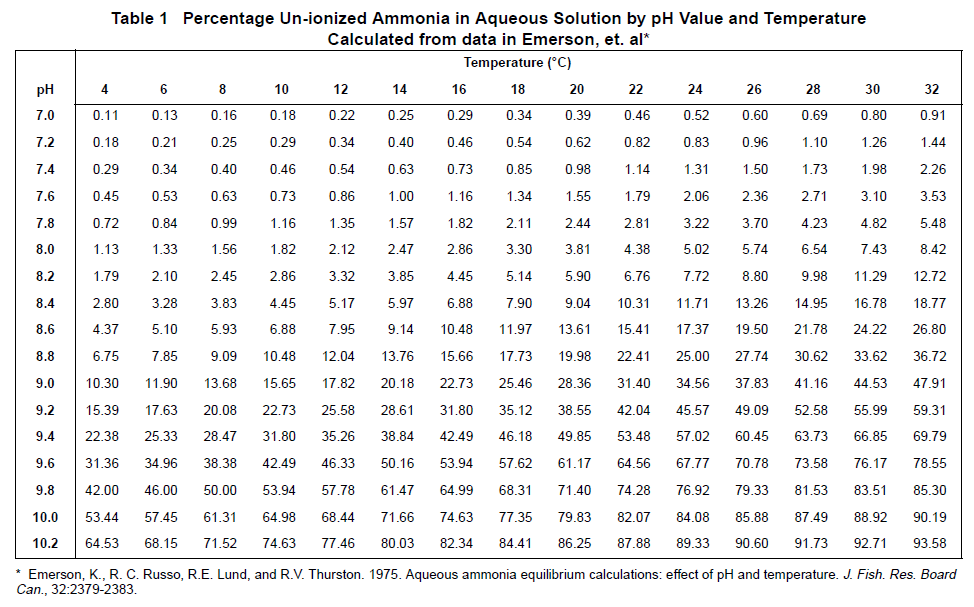Ammonia vs. Ammonium – what is the difference between these forms of nitrogen?

Dokument-ID

Dokument-ID TE2796

Veröffentlichungsdatum

Veröffentlichungsdatum 18.08.2020
Frage
Ammonia vs. Ammonium – what is the difference between these forms of nitrogen?
Zusammenfassung
Ammonia vs. Ammonium
Antwort
Ammonia and ammonium are different forms of nitrogen. The major factor that determines the proportion of ammonia to ammonium in water is pH. The activity of the ammonia is also influenced by ionic strength and temperature. It is important to remember as un‐ionized NH 3 can be harmful to aquatic organisms, while ionized ammonium is basically harmless.

The chemical equation that drives the relationship between ammonia and ammonium is:
NH 3 + H2O <-> NH 4 + + OH -

When the pH is low, the reaction is driven to the right, and when the pH is high, the reaction is driven to the left. In general, at a temperature of around room temperature, at a pH less than 6.0, the proportion of ammonium‐N plus ammonia‐N as NH 3 is very‐very low and as NH 4+ is very‐very high. At a pH around 8.0, the proportion as NH 3 is 10 percent or less, and at a pH slightly above 9.0, the proportion is about 50 percent. The activity of aqueous ammonia also is much lower at low temperatures and higher at warm temperatures. This means that at low temperatures and low pH the activity as NH 3 is even lower, and as NH 4+ is even higher. Once the pH is > 11, all ammonium‐N ions in solution will be converted to ammonia‐N.

The IntelliCAL™ ISENH3181 Ammonia ISE can be used to measure the ammonium ion (NH 4+ ) after converting to ammonia (NH 3). This is done through addition of the Hach Ammonia ISA, which buffers the pH to > 11 and increases the ionic strength of the sample. The Ammonia ISE method measures ammonium‐N plus ammonia‐N. It is very difficult to directly determine the activity of aqueous ammonia, so instead the surrogate of ammonium‐N plus ammonia‐N is used, and then tabled values of ammonium‐ N plus ammonia‐N are used to determine if a measured concentration will provide ammonia at a level that is detrimental to aquatic organisms.

If the sample temperature and sample pH are known, the below table can be used to determine what percentage of total ammonia is in the ammonia (NH 3) form. This percentage in decimal form can be multiplied by the total ammonia of the sample to calculate the NH 3 form ammonia. The difference between the total ammonia and the NH 3 form ammonia can be used to calculate the ammonium ion (NH 4+ ) is in the sample.